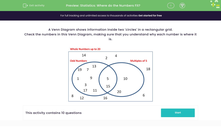# Statistics: Understand Venn diagrams

In this worksheet, students answer questions related to a diagram.Key stage:  KS 2

Curriculum topic:   Statistics

Curriculum subtopic:   Compare Data Using Charts and Graphs

Difficulty level:#### Worksheet Overview

This Venn Diagram shows information inside two 'circles' in a rectangular grid.

Check the numbers in this Venn Diagram, making sure that you understand why each number is where it is.

The numbers in the left hand 'circle' are odd

The numbers in the right hand 'circle' are multiples of 5

The numbers in the section where the 'circles' overlap are both odd and multiples of 5

Numbers outside the circles are whole numbers up to 20, that are neither odd nor multiples of 5### What is EdPlace?

We're your National Curriculum aligned online education content provider helping each child succeed in English, maths and science from year 1 to GCSE. With an EdPlace account you’ll be able to track and measure progress, helping each child achieve their best. We build confidence and attainment by personalising each child’s learning at a level that suits them.

Get started